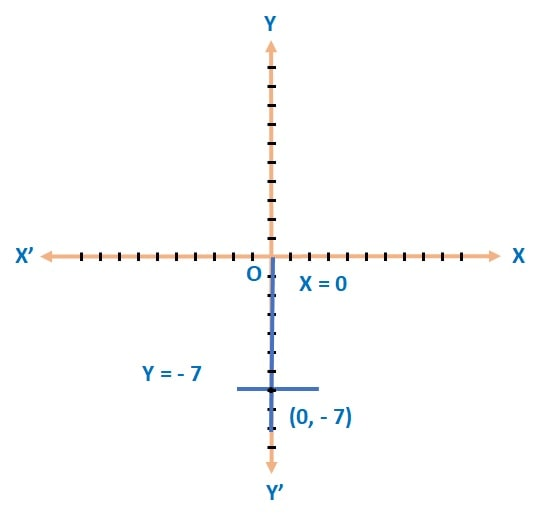Q) Using graphical method, find whether following system of linear equations x = 0 and y = – 7 is consistent or not.

Ans:Draw graph for y = – 7 and x = 0.

As y = – 7 is intersecting x = 0 at (0,-7);

Therefore, system of equations is consistent.

Scroll to Top